The QLIM Procedure

Ordinal Discrete Choice Modeling

Subsections:

Binary Probit and Logit Model

The binary choice model is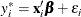where value of the latent dependent variable,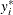, is observed only as follows: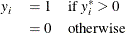The disturbance,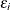, of the probit model has standard normal distribution with the distribution function (CDF)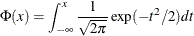The disturbance of the logit model has standard logistic distribution with the CDF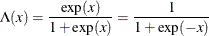The binary discrete choice model has the following probability that the event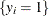occurs: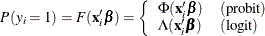The log-likelihood function is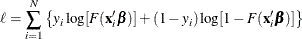where the CDF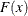is defined as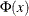for the probit model while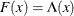for logit. The first order derivatives of the logit model are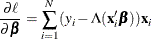The probit model has more complicated derivatives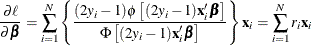where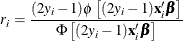Note that the logit maximum likelihood estimates are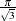times greater than probit maximum likelihood estimates, since the probit parameter estimates,, are standardized, and the error term with logistic distribution has a variance of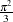.

Ordinal Probit/Logit

When the dependent variable is observed in sequence with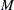categories, binary discrete choice modeling is not appropriate for data analysis. McKelvey and Zavoina (1975) proposed the ordinal (or ordered) probit model.

Consider the following regression equation: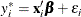where error disturbances,, have the distribution function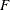. The unobserved continuous random variable,, is identified ascategories. Suppose there are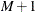real numbers,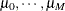, where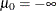,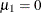,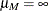, and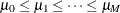. Define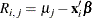The probability that the unobserved dependent variable is contained in the jth category can be written as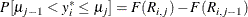The log-likelihood function is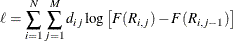where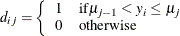The first derivatives are written as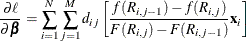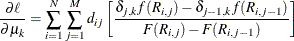where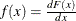and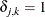if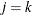, and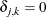otherwise. When the ordinal probit is estimated, it is assumed that. The ordinal logit model is estimated if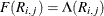. The first threshold parameter,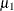, is estimated when the LIMIT1=VARYING option is specified. By default (LIMIT1=ZERO), so that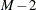threshold parameters (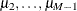) are estimated.

The ordered probit models are analyzed by Aitchison and Silvey (1957), and Cox (1970) discussed ordered response data by using the logit model. They defined the probability thatbelongs to jth category as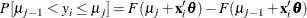whereand. Therefore, the ordered response model analyzed by Aitchison and Silvey can be estimated if the LIMIT1=VARYING option is specified. Note that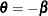.

Goodness-of-Fit Measures

The goodness-of-fit measures discussed in this section apply only to discrete dependent variable models.

McFadden (1974) suggested a likelihood ratio index that is analogous to the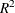in the linear regression model: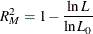where
is the value of the maximum likelihood function and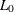is the value of a likelihood function when regression coefficients except an intercept term are zero. It can be shown thatcan be written as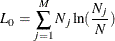where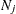is the number of responses in category j.

Estrella (1998) proposes the following requirements for a goodness-of-fit measure to be desirable in discrete choice modeling:

• The measure must take values in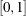, where 0 represents no fit and 1 corresponds to perfect fit.

• The measure should be directly related to the valid test statistic for significance of all slope coefficients.

• The derivative of the measure with respect to the test statistic should comply with corresponding derivatives in a linear regression.

Estrella’s (1998) measure is written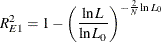An alternative measure suggested by Estrella (1998) is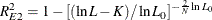where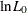is computed with null slope parameter values,
is the number observations used, and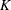represents the number of estimated parameters.

Other goodness-of-fit measures are summarized as follows: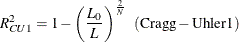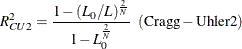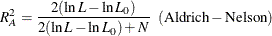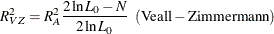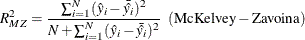where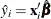and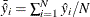.